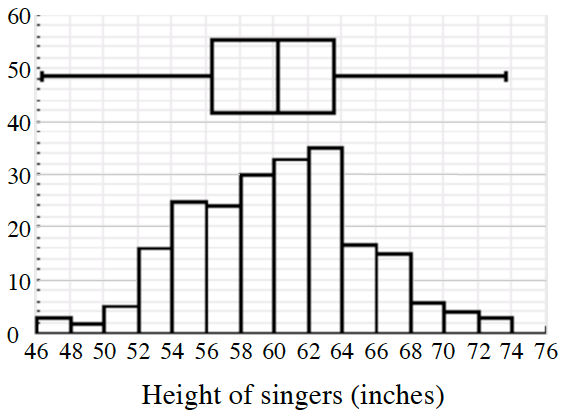### Home > INT3 > Chapter 4 > Lesson 4.4.3 > Problem4-126

4-126.

Mrs. Bell measures the heights of the $218$ singers in the girls’ chorus so she can place them on the risers with shorter girls in front and taller girls in back. A calculator screen shot of the distribution of the heights is shown at right.

1. In approximately what percentile is a singer who is $4'9''$tall?

Each bar of the graph represents an amount of singers. Add up the heights of the bars on the graph for singers under $57''$ tall and divide by $218$.

2. Mrs. Bell models the heights of her singers using a normal distribution with a mean of $60.2$ inches and a standard deviation of $5.2$. She assumes her model applies to all $1450$ girls at the state competition. How many singers at the competition can Mrs. Bell expect to be taller than $5'10''$?

Use your calculator. What are the lower and upper bounds of the heights you are interested in?

normalcdf($70$, $10$^$99$, $60.2$, $5.2$)